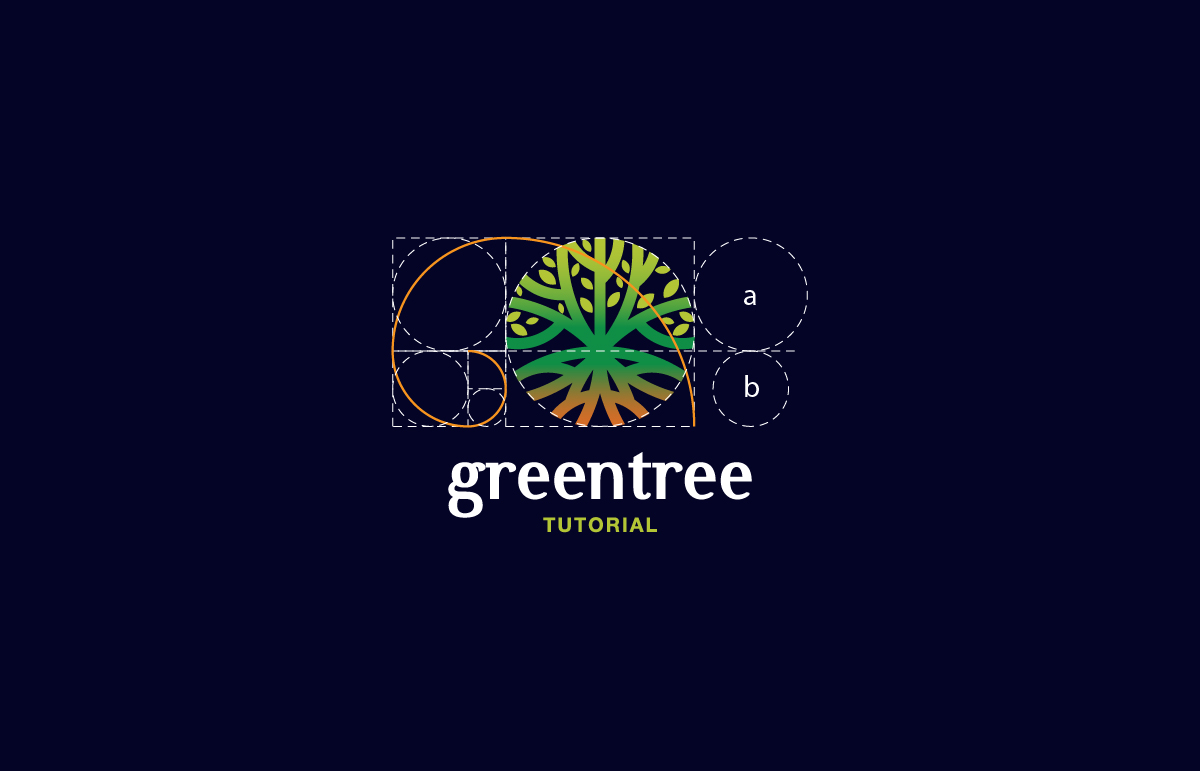What Is The Golden Ratio?

The Golden Ratio is a mathematical ratio. It is commonly found in nature, and it can also be used to achieve beauty, balance and harmony in art, design ( such as: architecture, logos, UI Design…) and photography. It is a special number found by dividing a line into two parts so that the longer part divided by the smaller part is also equal to the whole length divided by the longer part.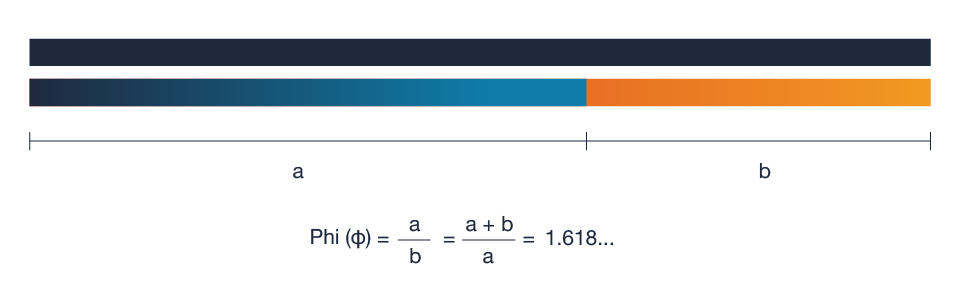What is the Golden Ratio?

A Fibonacci sequence starting with 0 and 1. The previous two numbers are added together to produce the next number in the sequence: 0,1,1, 2, 3, 5, 8, 13, 21, …  and so on to infinity.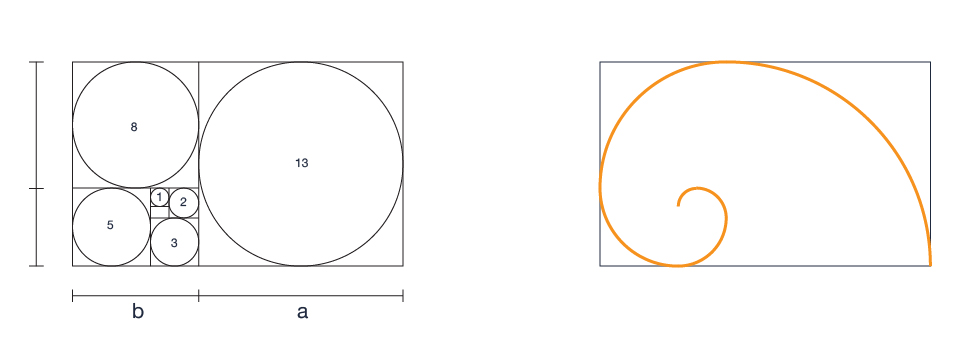Golden ratio Number

## Here are some examples of logos designed according to the Golden Ratio by Dainogo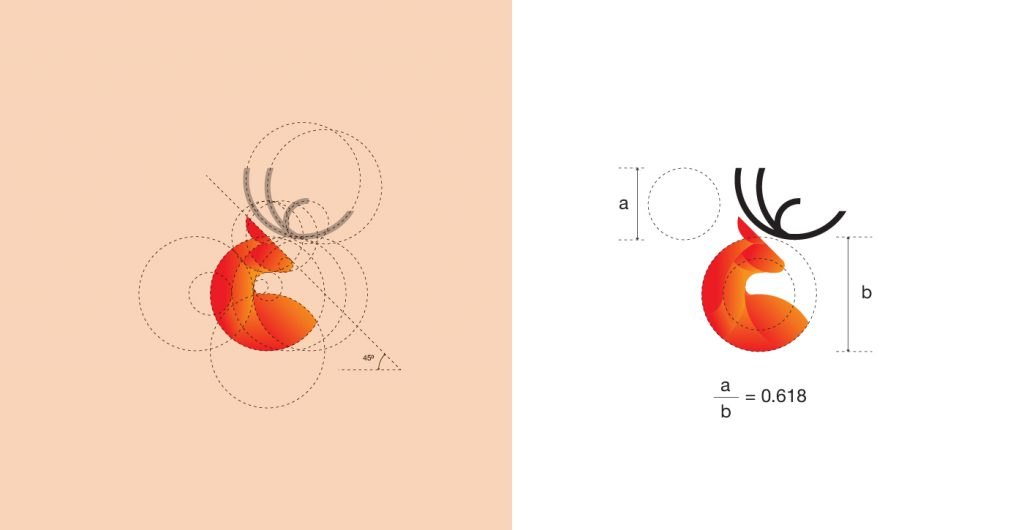Deer Logo Design based on Golden Ratio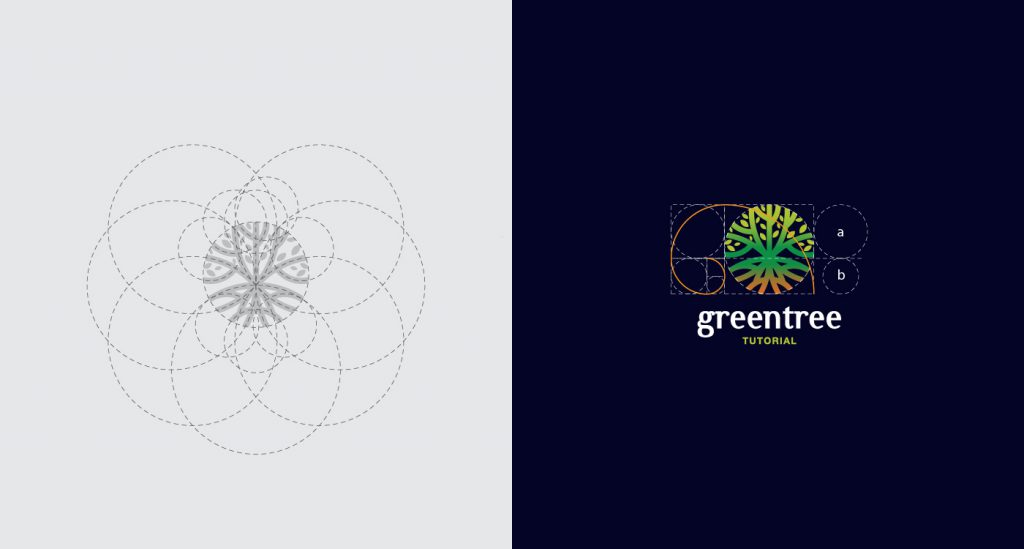Green Tree Logo Design based on Golden Ratio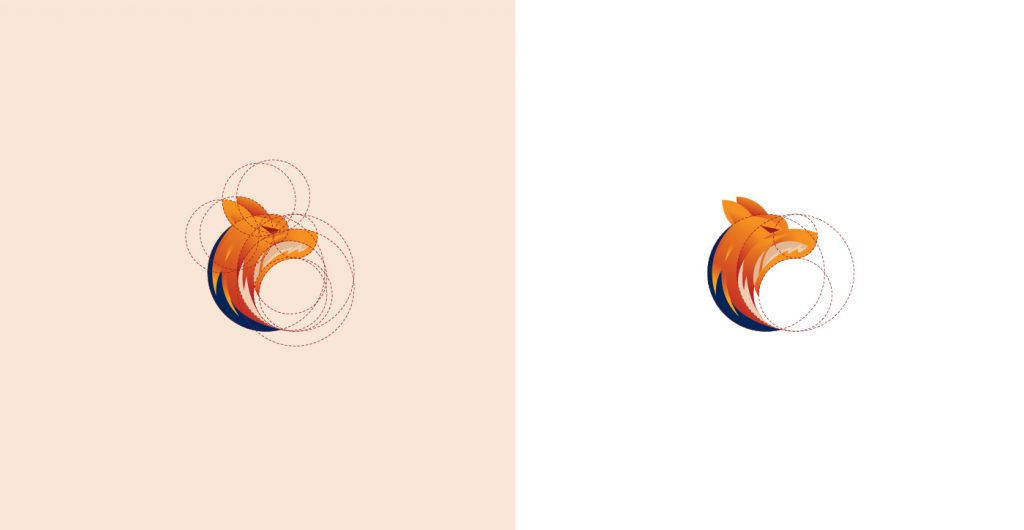Fox Logo Design based on Golden Ratio

## How to create Golden Ratio grid?

Let’s start by creating a golden rectangle. To make this simple, i’ll start with a width of 1000 pixels and height is 618 pixels. Then add a 618 x 618 square on the right side of the canvas, then a 382x 382 square… and so on.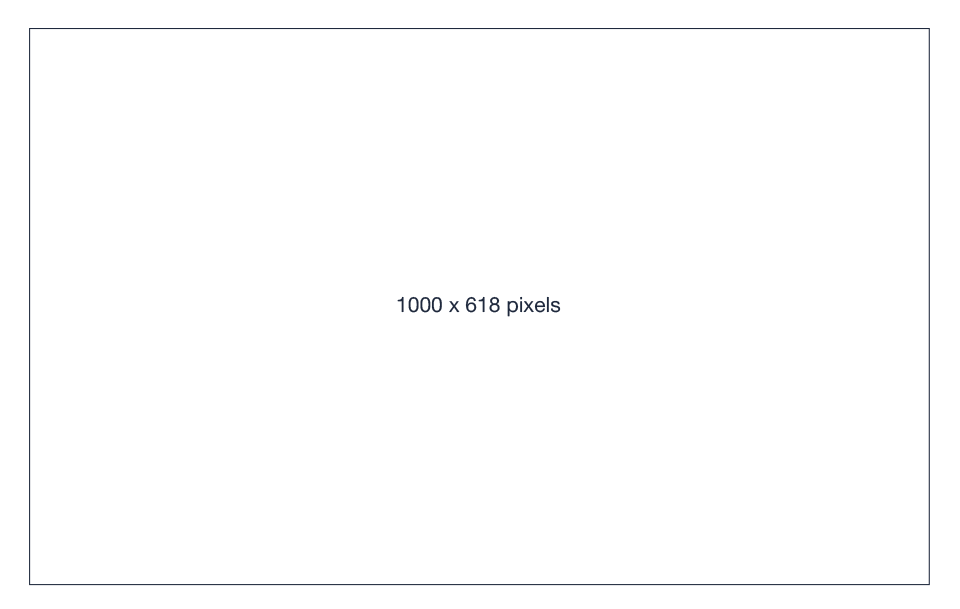Create grid of Golden Ratio

Other blogs: Sach hay 2019

1.2.